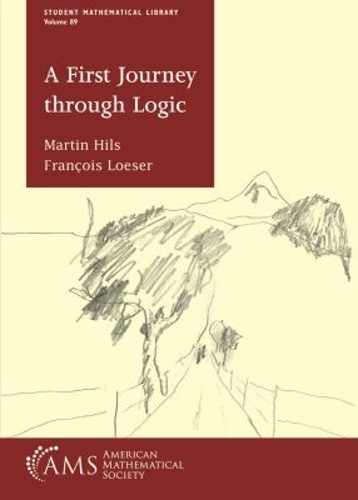•# A First Journey through Logic - Student Mathematical Library (Paperback)

(author), (author)
£52.95
Paperback 195 Pages / Published: 30/10/2019
• We can order this

Usually dispatched within 2 weeks

The aim of this book is to present mathematical logic to students who are interested in what this field is but have no intention of specializing in it. The point of view is to treat logic on an equal footing to any other topic in the mathematical curriculum. The book starts with a presentation of naive set theory, the theory of sets that mathematicians use on a daily basis. Each subsequent chapter presents one of the main areas of mathematical logic: first order logic and formal proofs, model theory, recursion theory, Godel's incompleteness theorem, and, finally, the axiomatic set theory. Each chapter includes several interesting highlights--outside of logic when possible--either in the main text, or as exercises or appendices. Exercises are an essential component of the book, and a good number of them are designed to provide an opening to additional topics of interest.

Publisher: American Mathematical Society
ISBN: 9781470452728
Number of pages: 195
Dimensions: 216 x 140 mm